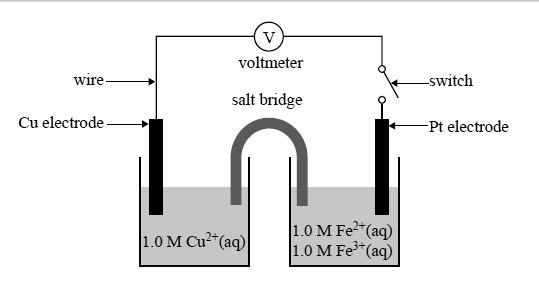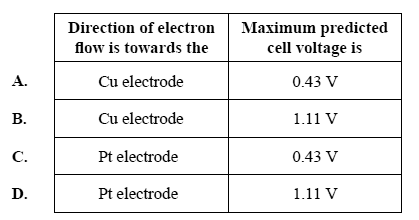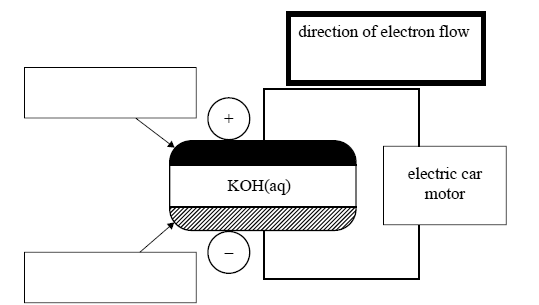Galvanic cells (2015 VCE) 1) The reaction between hydrogen peroxide and ammonium ions is represented by the following equation. 3H2O2(aq) + 2NH4+(aq) => N2(g) + 2H+(aq) + 6H2O(l) Which one of the following is the correct half-equation for the reduction reaction? A. H2O2(aq) + 2H+(aq) + 2e– => 2H2O(l) B. 2NH4+(aq) => N2(g) + 8H+(aq) + 6e– C. 2NH4+(aq) + 2e– =>N2(g) + 4H2(g) D. H2O2(aq) + 2H2O(l)=> 2O2(g) + 6H+(aq) + 6e– Solution2) Solution I – 1.0 M NaCl Solution II – 1.0 M CuCl2 Solution III – 1.0 M MgCl2 Which solution or solutions above will react with Zn powder? A. Solution I only B. Solution II only C. Solutions I and III only D. Solutions I, II and III Solution3) The switch in the galvanic cell below may be closed to allow a current to fl ow through the circuit.Which of the following best describes the direction of electron fl ow in the external circuit or wire, and the maximum predicted cell voltage measured at the voltmeter when the switch is closed?Solution4) Which one of the following classes of electrochemical cells involves only a non-spontaneous redox reaction? A. fuel cells B. electroplating cells C. primary galvanic cells D. secondary galvanic cells   Solution5) The storage battery to be used in the hybrid cars is comprised of a series of nickel metal hydride, NiMH, cells. MH represents a metal hydride alloy that is used as one electrode. The other electrode contains nickel oxide hydroxide, NiOOH. The electrolyte is aqueous KOH.The simplified equation for the reaction at the anode while recharging is Ni(OH)2(s) + OH–(aq)  NiOOH(s) + H2O(l) + e– The simplified equation for the reaction at the cathode while recharging is M(s) + H2O(l) + e–  MH(s) + OH–(aq) a. What is the overall equation for the discharging reaction? Solutionb. i. In the boxes on the diagram above, indicate which is the MH electrode and which is the NiOOH electrode. ii. In the bold box provided above the cell diagram, use an arrow, <- or ->, to indicate the direction of the electron flow as the cell is discharging. Solutionc. The battery discharged for 60 minutes, producing a current of 1.15 A. What mass, in grams, of NiOOH would be used during this period? Solution